First we load the R/qtl package and print the version we’re using.

``````> library(qtl)
> qtlversion()
 "1.25-15"``````

We load the raw genotype data, which includes data on the parental strains and F1. We drop the control mouse with a blank for “strain.”

``````> rawg <- read.cross("csv", "Data", "genotypes4rqtl.csv", genotypes=0:2,
+                    convertXdata=FALSE, alleles=c("B","R"))
> rawg <- subset(rawg, ind=(rawg\$pheno\$Strain!=""))``````

The mouse with missing strain also had missing sex, we clean up the sex and strain phenotypes as follows:

``````> rawg\$pheno\$Sex <- factor(as.character(rawg\$pheno\$Sex), levels=c("Female", "Male"))
> rawg\$pheno\$Strain <- factor(as.character(rawg\$pheno\$Strain), levels=unique(as.character(rawg\$pheno\$Strain)))``````

A quick summary:

``````> summary(rawg)
F2 intercross

No. individuals:    565

No. phenotypes:     5
Percent phenotyped: 100 100 100 100 100

No. chromosomes:    21
Autosomes:      1 2 3 4 5 6 7 8 9 10 11 12 13 14 15 16 17 18 19 un
X chr:          X

Total markers:      4853
No. markers:        386 343 340 282 256 274 234 248 216 219 257 218 230 240 197 243 183 194 117 169 7
Percent genotyped:  98.6
Genotypes (%):      BB:40  BR:21.3  RR:38.8  not RR:0  not BB:0``````

Note that the chromosome `"un"` is a group of 7 markers that I couldn’t find in the mouse genome build 37.

## 2. Missing data pattern

We first look at the number of missing genotypes at each mouse and each marker.

``````> par(mfrow=c(2,1), las=1)
> hist(nmissing(rawg), main="No. missing genotypes by mouse",
+      xlab="No. missing genotypes", breaks=140)
> rug(nmissing(rawg), col="blue")
> hist(nmissing(rawg, what="mar"), main="No. missing genotypes by marker",
+      xlab="No. missing genotypes", breaks=50)
> rug(nmissing(rawg, what="mar"), col="blue")``````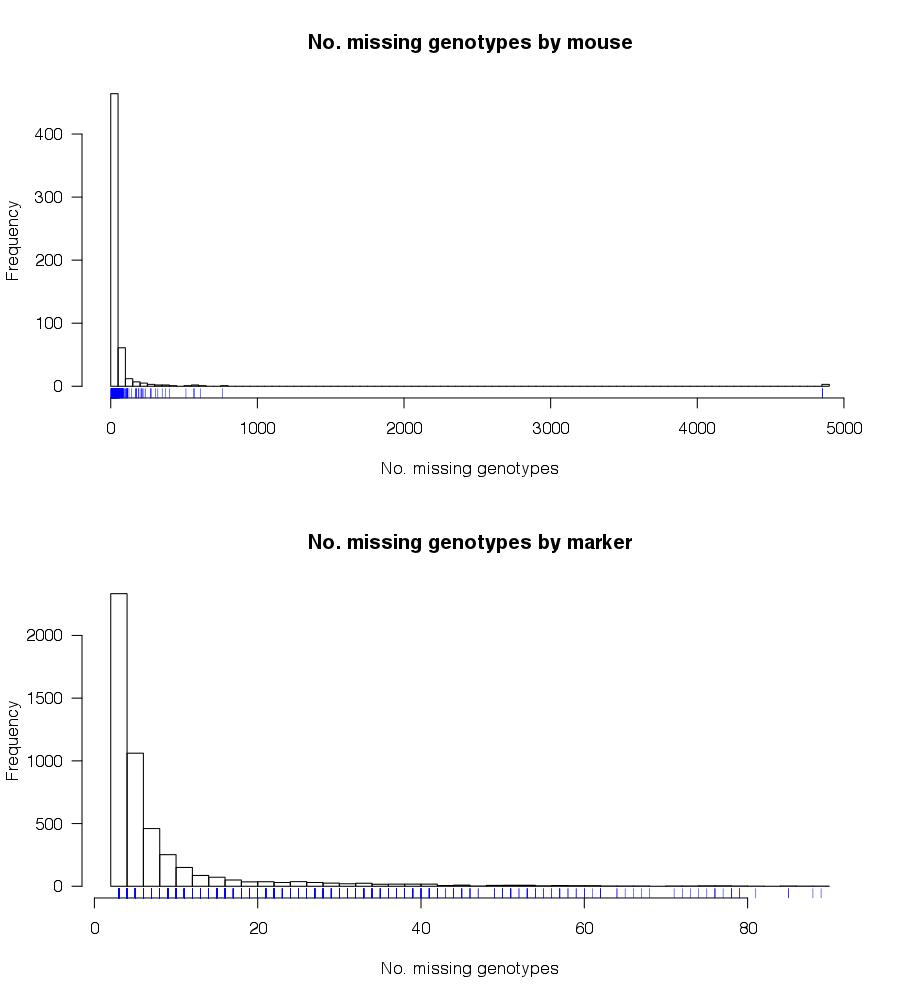There are three mice with no genotype data.

``````> rawg\$pheno[ntyped(rawg)==0,]
longid  MouseNum    Sex Strain         PlatePos
265   tid28116_Mouse3377_F2_Male Mouse3377   Male     F2 SMP4_0001630:C04
311   tid28077_Mouse3338_F2_Male Mouse3338   Male     F2 SMP4_0001632:B10
498 tid28004_Mouse3265_F2_Female Mouse3265 Female     F2 SMP4_0001632:A01``````

I might as well drop these.

``> rawg <- subset(rawg, ind=(ntyped(rawg)>0))``

We should look at the histogram of the number of missing genotypes per mouse again.

``````> par(las=1)
> hist(nmissing(rawg), main="No. genotypes by mouse",
+      xlab="No. markers genotyped", breaks=140)
> rug(nmissing(rawg), col="blue")``````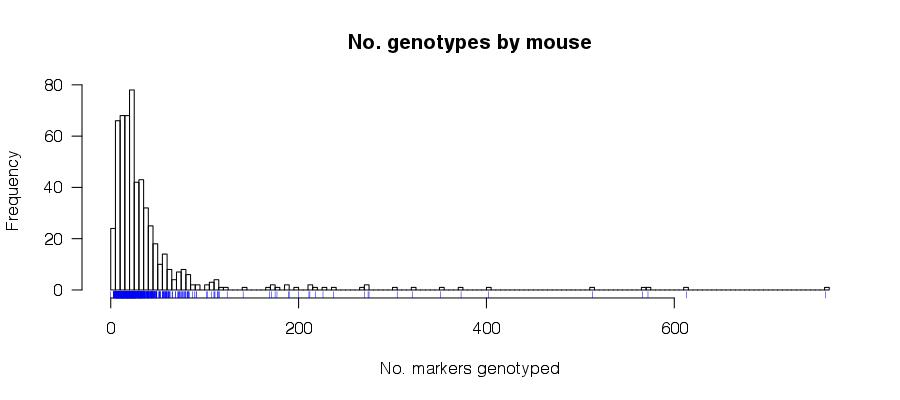There remain a number of mice with a good amount of missing data, which might suggest poor-quality DNAs or other problems with the genotyping arrays. There are 13 mice missing genotypes at more than 5% of the markers (that is, at more than 242 of the 4853 markers). There are also a number of markers with elevated rates of missing data. There are 188 markers with more than 5% missing data (that is, at more than 28 of the 562 mice). But none of these seem terrible.

## 3. Study X chromosome genotypes

Let’s start by looking at the X chromosome genotypes. The cross was (BTBR x B6) x (BTBR x B6), with females listed first, and so the F1 males should all be hemizygous B6 or BTBR while the F1 females should be heterozygous. The F2 males should be hemizygous B6 or BTBR and the F2 females should be heterozygous or homozygous BTBR.

We pull out the X chromosome genotypes and the “strain” and sex for each mouse.

``````> gx <- pull.geno(rawg, chr="X")
> dim(gx)
 562 169
> strain <- rawg\$pheno\$Strain
> sex <- rawg\$pheno\$Sex``````

### 3.1. Not segregating

First, let’s look at which markers seem to segregate in the F2. We create a table of the three possible genotypes (1, 2, 3) and look at the number of mice with the least-frequent genotype. Since there are 289 F2 males and 262 F2 females, all markers should have an appreciable number of mice with each of the three genotypes.

``````> ming <- apply(apply(gx[strain=="F2",], 2, function(a) table(factor(a, levels=1:3))), 2, min)
> table(ming)
ming
0   1   2   3   6 120 125 126 131 132 133 134 136 138 143
138   6   2   2   1   1   2   1   5   2   3   3   1   1   1``````

There are 149 markers that appear to not be segregating. Let’s go ahead and omit those. We’ll create a new cross object `cleang` that will contain the cleaned genotypes.

``````> todrop <- names(ming[ming<120])
> cleang <- drop.markers(rawg, todrop)
> gx <- pull.geno(cleang, chr="X")
> dim(gx)
 562  20``````

We have just 20 markers left on the X chromosome.

### 3.2. Genotypes in B6 and BTBR

Let’s first look at the B6 mice. There are 3 such; while 2 are male and 1 is female, they should all be homozygous for the same allele.

``````> all(apply(gx[strain=="B6",], 2, function(a) length(unique(a[!is.na(a)]))) == 1)
 TRUE
> b6xg <- apply(gx[strain=="B6",], 2, function(a) unique(a[!is.na(a)]))``````

All of the B6 mice are homozygous for the same allele, on the X chromosome.

We repeat this for the 4 BTBR mice, of which 2 are male and 2 are female.

``````> all(apply(gx[strain=="BTBR",], 2, function(a) length(unique(a[!is.na(a)])) == 1))
 TRUE
> btbrxg <- apply(gx[strain=="BTBR",], 2, function(a) unique(a[!is.na(a)]))``````

All of the BTBR mice are homozygous for the same allele, on the X chromosome.

We further check that the B6 and BTBR genotypes are really homozygous and for different alleles at these 20 markers.

``````> all((b6xg == 1 & btbrxg==3) | (b6xg == 3 & btbrxg==1))
 TRUE``````

Let’s swap the genotype codes for the markers where the B6 mice have the `3` genotype (to make it so that `1` is homozygous B6 and `3` is homozygous BTBR).

``````> toswap <- names(b6xg)[b6xg==3]
> for(mar in toswap)
+   cleang\$geno[["X"]]\$data[,mar] <- 4 - cleang\$geno[["X"]]\$data[,mar]
> gx <- pull.geno(cleang, chr="X")``````

### 3.3. Genotypes in F1

Let’s look at the 4 F1 mice, of which 2 are male and 2 are female. The males should all by homozygous BTBR and the females should all by heterozygous.

``````> f1male.gx <- gx[strain=="F1" & sex=="Male",]
> f1female.gx <- gx[strain=="F1" & sex=="Female",]
> all(is.na(f1male.gx) | f1male.gx==3)
 TRUE
> all(is.na(f1female.gx) | f1female.gx==2)
 TRUE``````

Everything looks good.

### 3.4. Genotypes in F2 males

We now turn to the F2 males. They should all be hemizgous B6 (`1`) or BTBR (`3`).

``````> f2male.gx <- gx[strain=="F2" & sex=="Male",]
> sum(!is.na(f2male.gx) & f2male.gx==2)
 171``````

There are 171 heterozygous genotypes in the 289 male mice. Let’s look at the males that have heterozygous calls.

``````> wh <- apply(f2male.gx, 1, function(a) any(!is.na(a) & a==2))
> sum(wh)
 18
> tab <- apply(f2male.gx[wh,], 1, function(a) table(factor(a[!is.na(a)], levels=1:3)))
> tab[,order(tab[2,])]
[,1] [,2] [,3] [,4] [,5] [,6] [,7] [,8] [,9] [,10] [,11] [,12] [,13] [,14] [,15] [,16] [,17] [,18]
1    7    5   13   15    0    0    0    0    0     0     0     0     0     0     0     0     0     0
2    1    1    1    1    4    4    5    7    8    10    11    13    14    15    16    20    20    20
3   12   13    5    3   15   16   14   13   12     9     9     7     6     5     4     0     0     0``````

The first 4 of these look to be male but with a single genotyping error. The other 14 look to be really female. Let’s save the IDs for these mice.

``````> malef2id <- as.character(cleang\$pheno\$MouseNum)[strain=="F2" & sex=="Male"]
> notmale <- malef2id[apply(f2male.gx, 1, function(a) sum(!is.na(a) & a==2)>1)]``````

Note that there are additional male mice that might really be females; if all of their genotypes on the X chromosome are homozygous/hemizygous BTBR, we can’t tell.

``````> sum(apply(f2male.gx, 1, function(a) all(is.na(a) | a==3)))
 50``````

### 3.5. Genotypes in F2 females

We turn to the F2 females. They should all be heterozygous (`2`) or homozygous BTBR (`3`).

``````> f2female.gx <- gx[strain=="F2" & sex=="Female",]
> sum(!is.na(f2female.gx) & f2female.gx==1)
 270``````

There are 270 homozygous B6 genotypes in the 262 female mice. Let’s look at the females that have homozygous B6 calls.

``````> wh <- apply(f2female.gx, 1, function(a) any(!is.na(a) & a==1))
> sum(wh)
 21
> tab <- apply(f2female.gx[wh,], 1, function(a) table(factor(a[!is.na(a)], levels=1:3)))
> tab[,order(tab[1,])]
[,1] [,2] [,3] [,4] [,5] [,6] [,7] [,8] [,9] [,10] [,11] [,12] [,13] [,14] [,15] [,16] [,17] [,18] [,19] [,20] [,21]
1    5    5    5    7    8    9   10   11   11    13    13    13    15    16    16    16    18    19    20    20    20
2    0    0    0    0    0    0    0    0    0     0     0     0     0     0     0     0     0     0     0     0     0
3   15   15   15   13    8   10   10    9    9     7     7     7     5     4     4     4     2     0     0     0     0``````

These all look to be clearly males. Let’s save the IDs for these mice.

``````> femalef2id <- as.character(cleang\$pheno\$MouseNum)[strain=="F2" & sex=="Female"]
> notfemale <- femalef2id[apply(f2female.gx, 1, function(a) sum(!is.na(a) & a==1)>1)]``````

Note that there are additional female mice that might really be males; if all of their genotypes on the X chromosome are homozygous/hemizygous BTBR, we can’t tell.

``````> sum(apply(f2female.gx, 1, function(a) all(is.na(a) | a==3)))
 53``````

### 3.6. Swap sexes

Let’s go ahead and swap the sexes of these mice whose X chromosome genotypes indicate they are the opposite sex. We’ll change the phenotype `Sex` to `SexID` and make a new “phenotype” `Sex`.

``````> names(cleang\$pheno)[names(cleang\$pheno)=="Sex"] <- "SexID"
> cleang\$pheno\$Sex <- cleang\$pheno\$SexID
> cleang\$pheno\$Sex[match(notmale, cleang\$pheno\$MouseNum)] <- "Female"
> cleang\$pheno\$Sex[match(notfemale, cleang\$pheno\$MouseNum)] <- "Male"
> table(cleang\$pheno\$SexID, cleang\$pheno\$Sex)
Female Male
Female    246   21
Male       14  281``````

I’ll re-create my `sex` vector to match the new values.

``> sex <- cleang\$pheno\$Sex``

### 3.7. Re-code genotypes

Now let’s recode the X chromosome genotypes. F2 females should have genotype codes `1` for homozygous BTBR and `2` for heterozygous. F2 males should have genotype codes `1` for homozygous B6 and `2` for homozygous BTBR.

First, we handle the males.

``````> gx <- pull.geno(cleang, chr="X")
> whmale <- strain=="F2" & sex=="Male"
> gxmale <- gx[whmale,]
> sum(!is.na(gxmale) & gxmale==2)
 4
> gxmale[!is.na(gxmale) & gxmale==2] <- NA
> gxmale[!is.na(gxmale) & gxmale==3] <- 2
> gx[whmale,] <- gxmale``````

Now, we handle the females.

``````> whfemale <- strain=="F2" & sex=="Female"
> gxfemale <- gx[whfemale,]
> sum(!is.na(gxfemale) & gxfemale==1)
 0
> gxfemale[!is.na(gxfemale) & gxfemale==1] <- NA
> gxfemale[!is.na(gxfemale) & gxfemale==3] <- 1
> gx[whfemale,] <- gxfemale``````

Now we paste the genotypes back into the cross object.

``> cleang\$geno[["X"]]\$data <- gx``

Let’s further add a `pgm` phenotype, indicating cross direction, which will be 1 for all F2 mice.

``````> cleang\$pheno\$pgm <- rep(NA, nind(cleang))
> cleang\$pheno\$pgm[strain=="F2"] <- 1``````

The summary of the cross (with just the F2 mice) should be clean. (But then, the warnings don’t get printed here anyway!)

``````> summary(subset(cleang, ind=(strain=="F2")))
F2 intercross

No. individuals:    551

No. phenotypes:     7
Percent phenotyped: 100 100 100 100 100 100 100

No. chromosomes:    21
Autosomes:      1 2 3 4 5 6 7 8 9 10 11 12 13 14 15 16 17 18 19 un
X chr:          X

Total markers:      4704
No. markers:        386 343 340 282 256 274 234 248 216 219 257 218 230 240 197 243 183 194 117 20 7
Percent genotyped:  99.1
Genotypes (%):      BB:39.6  BR:22.2  RR:38.3  not RR:0  not BB:0``````

### 3.8. Segregation patterns

Let’s look at the segregation of the genotypes on the X chromosome. First, let’s look at the output of geno.table. What we’re looking for here are departures from 1:1 segregation that might indicate markers with high rates of genotyping errors.

``````> geno.table(subset(cleang, ind=(strain=="F2")), chr="X")
chr missing BB  BR  RR not.RR not.BB  BY  RY P.value
rs13483704   X       2  0 120 134      0      0 150 145  0.6517
rs13483715   X       1  0 125 130      0      0 149 146  0.9377
rs13483716   X       3  0 125 128      0      0 150 145  0.9416
rs13483718   X       0  0 126 129      0      0 155 141  0.7056
rs13483737   X       1  0 131 123      0      0 151 145  0.8296
rs13483738   X       1  0 131 124      0      0 149 146  0.8946
rs13483741   X       3  0 131 123      0      0 148 146  0.8757
rs13483746   X       1  0 134 121      0      0 147 148  0.7167
rs13483750   X      19  0 132 120      0      0 146 134  0.5811
rs13483765   X       2  0 131 123      0      0 154 141  0.6620
rs13483766   X       7  0 132 122      0      0 152 138  0.5858
rs13483767   X       1  0 132 122      0      0 154 142  0.6440
rs13483770   X       1  0 134 121      0      0 148 147  0.7167
rs13484015   X      12  0 138 112      0      0 151 138  0.1931
rs13484018   X      25  0 141 107      0      0 144 134  0.0812
rs13459160   X       2  0 133 122      0      0 152 142  0.6654
rs3715531    X       3  0 133 122      0      0 150 143  0.7255
rs13484105   X       6  0 133 121      0      0 152 139  0.5634
rs13459161   X       3  0 131 124      0      0 147 146  0.9068
rs13484113   X       1  0 136 119      0      0 156 139  0.3477``````

Everything looks okay. Let’s make a couple of plots, of genotype frequencies along the X chromosome for each sex.

``````> par(mfrow=c(2,1), las=1)
> f2male <- subset(cleang, ind=(strain=="F2" & sex=="Male"))
> plot(geno.table(f2male, chr="X", scanone=TRUE), lod=8:9,
+      main="X chr genotype frequencies; F2 males",
+      ylab="Genotype frequency", col=c("blue","red"),
+      ylim=c(0.3, 0.7))
> abline(h=0.5, lty=2, col="gray")
> legend("topleft", lwd=2, col=c("blue","red"),
+        legend=c("hem B6", "hem BTBR"))
> f2female <- subset(cleang, ind=(strain=="F2" & sex=="Female"))
> plot(geno.table(f2female, chr="X", scanone=TRUE), lod=4:5,
+      main="X chr genotype frequencies; F2 females",
+      ylab="Genotype frequency", col=c("green", "red"),
+      ylim=c(0.3, 0.7))
> abline(h=0.5, lty=2, col="gray")
> legend("topleft", lwd=2, col=c("green","red"),
+        legend=c("het", "hom BTBR"))``````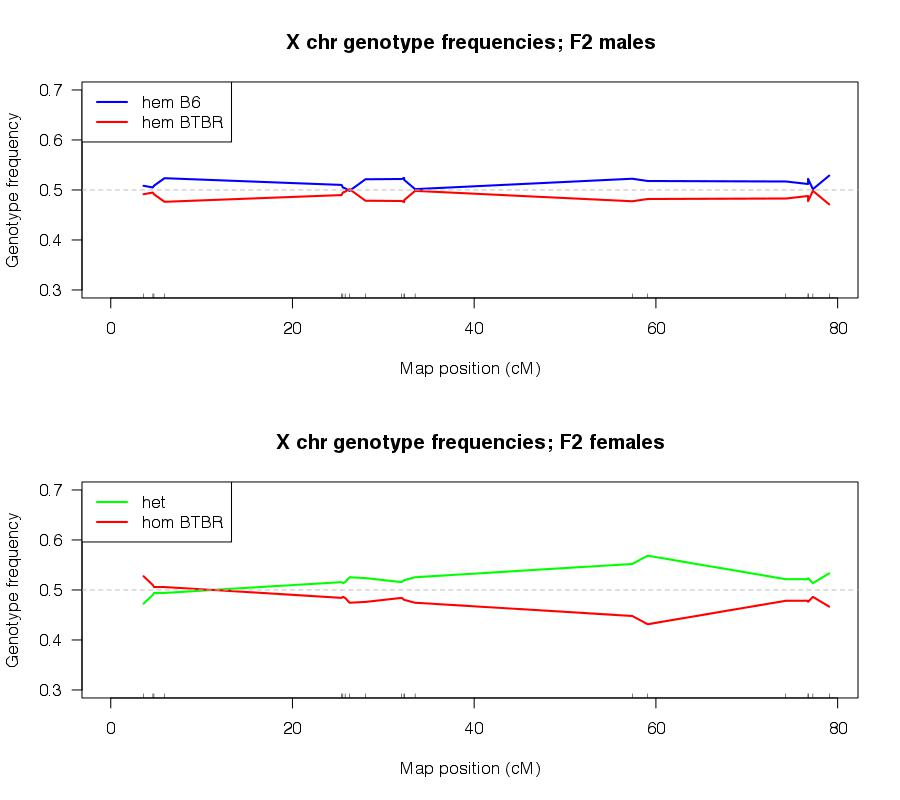The departures from 1:1 segregation seem not too worrisome.

### 3.9. Counts of crossovers

Let’s look at the number of crossovers in the F2 mice on the X chromosome.

``````> nxo <- countXO(subset(cleang, ind=(strain=="F2")), chr="X")
> table(nxo)
nxo
0   1   2   3   4   6
216 261  65   3   3   3``````

There are 9 mice with >2 crossovers. Let’s plot the genotype data for these mice. We run `calc.errorlod` to avoid a warning message. (The genotyping error LOD scores are used in `plot.geno` to flag potential genotyping errors.)

``````> temp <- subset(cleang, ind=(strain=="F2"))
> temp <- subset(temp, ind=(nxo>2), chr="X")
> temp <- calc.errorlod(temp, error.prob=0.002, map.function="c-f")
> plot.geno(temp, chr="X")``````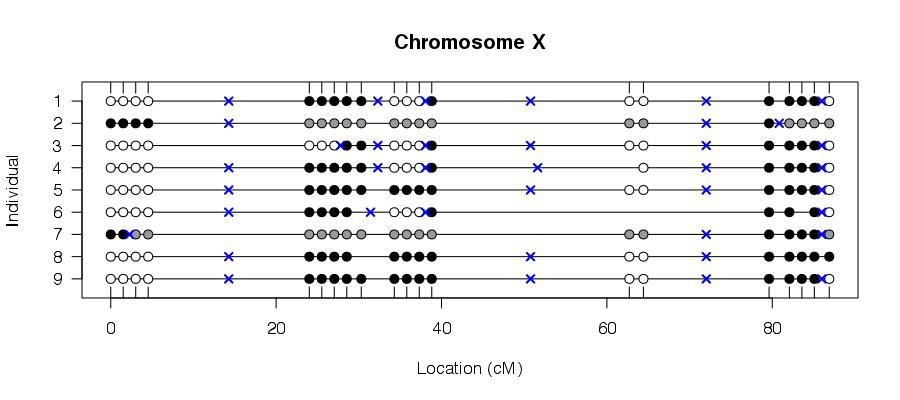These look unusual, but there are no obvious problems.

### 3.10. Re-estimate map

Finally, let’s re-estimate the genetic map for the X chromosome and see how it differs from the map from Cox et al. (Genetics, 182:1335-1344, 2009). I’ll use a genotype error rate of 2/1000, and the Carter-Falconer map function (which corresponds, approximately, to the high level of crossover interference in the mouse).

``````> f2g <- subset(cleang, ind=(strain=="F2"), chr="X")
> newmap <- est.map(f2g, err=0.002, map.function="c-f")``````

Let’s look at summaries of the original and the newly estimated maps.

``````> summary.map(f2g)[1,,drop=FALSE]
n.mar      length ave.spacing max.spacing
20.0        75.5         4.0        23.9
> summary.map(newmap)[1,,drop=FALSE]
n.mar      length ave.spacing max.spacing
20.0        77.7         4.1        24.0``````

Overall, the map hasn’t changed much.

Let’s plot the map next to the one embedded in the cross (which corresponds to that of Cox et al. 2009). The map on the bottom is the newly estimated one

``> plot.map(f2g, newmap, horizontal=TRUE)``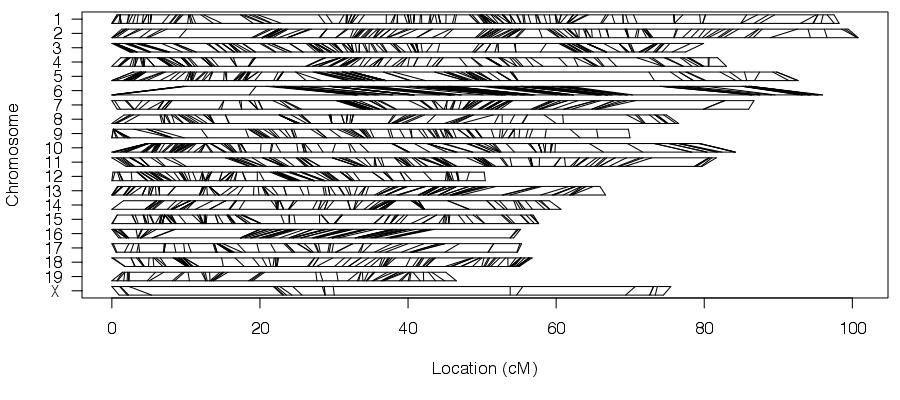It sure looks similar. It might be better to study the individual interval lengths.

``````> dom <- diff(pull.map(f2g)[])
> dnm <- diff(newmap[])
> par(las=1)
> plot(dom, dnm-dom, type="n", xlab="Original interval length (cM)",
+      ylab="Change in interval length (cM)")
> abline(h=0, lty=2, col="gray")
> text(dom, dnm-dom, seq(along=dnm))``````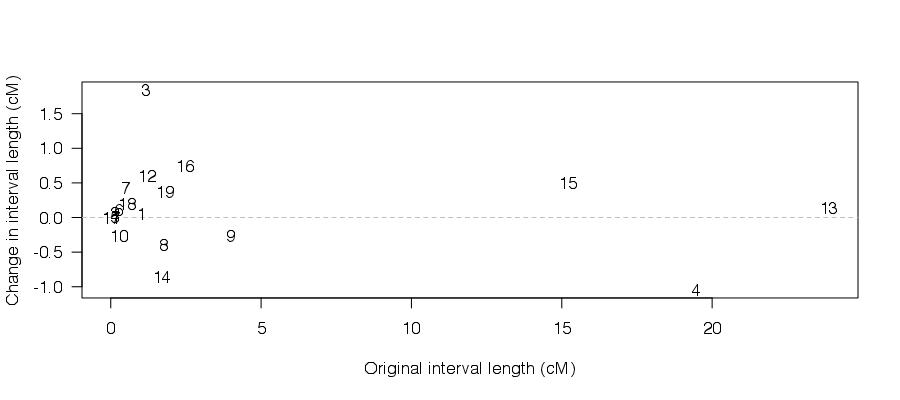Note that the numbers indicate the intervals. Some of the smaller intervals have changed a great deal in length, but overall it looks pretty good.

## 4. Study autosomal genotypes

We now turn to the autosomal genotypes.

``````> ga <- pull.geno(cleang, chr="-X")
> dim(ga)
  562 4684``````

### 4.1. Not segregating

First, let’s look at which markers seem to segregate in the F2. We create a table of the three possible genotypes (1, 2, 3) and look at the proportion of mice with the least-frequent genotype. Since there are 4684 markers, we’ll look at a histogram.

``````> ming <- apply(apply(ga[strain=="F2",], 2, function(a) table(factor(a, levels=1:3))/sum(!is.na(a))), 2, min)
> par(las=1)
> hist(ming, breaks=seq(0, ceiling(max(ming)*100)/100, by=0.005),
+      xlab="Frequency of least-frequent genotype", main="")
> rug(ming, col="blue")``````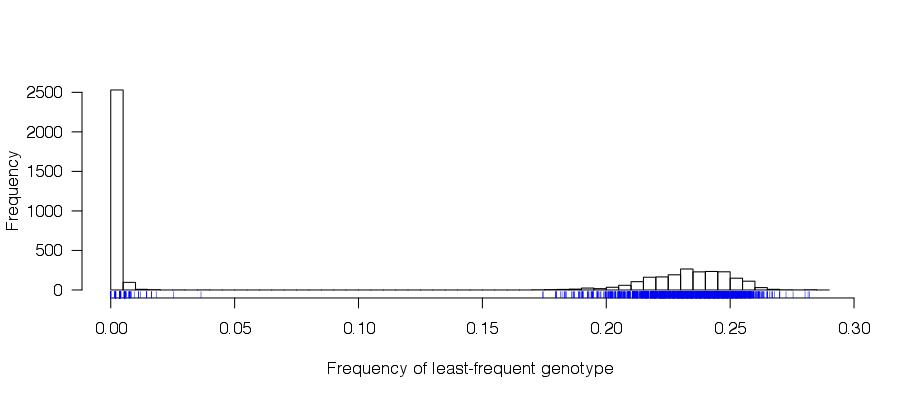Note the big gap between 4% and 17%. There are 2640 markers that appear not to be segregating, as the least-frequent genotype is present in <10% of mice. There are 2044 markers that do appear to be segregating, as the least-frequent genotype is present in >10% of mice.

Let’s go ahead and omit the non-segregating markers.

``````> todrop <- names(ming[ming<0.1])
> cleang <- drop.markers(cleang, todrop)
> ga <- pull.geno(cleang, chr="-X")
> dim(ga)
  562 2044``````

### 4.2. Genotypes in B6 and BTBR

Let’s look at the B6 mice. There are 3 such; they should all be homozygous for the same allele.

``````> bad.b6 <- apply(ga[strain=="B6",], 2, function(a) (length(unique(a[!is.na(a)]))) != 1 || all(is.na(a) | a==2))
 8
rs6163246 rs13478222 rs13478387 rs4226443 rs6188470 rs8259478 rs13481107 rs6358703
[1,]         3          2          2         2         3         2          2         2
[2,]         2         NA          3         3         2        NA          1         3
[3,]        NA         NA          3         3         2         3          1         3``````

There are 8 markers with heterozygous calls, including one with no homozygous calls.

Let’s grab the inferred genotype (`1`, `3`, or `NA`) for B6 mice.

``````> b6ga <- apply(ga[strain=="B6",], 2, function(a) if(all(is.na(a) | a==2)) return(NA) else return(unique(a[!is.na(a) & a!=2])))
> sum(is.na(b6ga))
 1
> table(b6ga)
b6ga
1    3
1036 1007``````

We repeat this for the 4 BTBR mice.

``````> bad.btbr <- apply(ga[strain=="BTBR",], 2, function(a) (length(unique(a[!is.na(a)]))) != 1 || all(is.na(a) | a==2))
 7
rs3022901 rs6183172 rs6173859 rs8259478 rs13481105 rs6302629 rs13483472
[1,]         1         1         1         1          1         3          2
[2,]         1         1         1         1          1         3          3
[3,]         1         1         1         1          1         3          3
[4,]         2         2         2         2          2         2          3``````

There are 7 markers with heterozygous calls.

Again, we pull out the inferred genotype for BTBR mice.

``````> btbrga <- apply(ga[strain=="BTBR",], 2, function(a) if(all(is.na(a) | a==2)) return(NA) else return(unique(a[!is.na(a) & a!=2])))
> sum(is.na(btbrga))
 0
> table(btbrga)
btbrga
1    3
1007 1037``````

We further check that the B6 and BTBR genotypes are homozygous for different alleles at these 2044 markers.

``````> all(is.na(b6ga) | (b6ga==1 & btbrga==3) | (b6ga==3 & btbrga==1))
 TRUE``````

Let’s swap the genotype codes for the markers where the BTBR mice have the `1` genotype (to make it so that `1` is homozygous B6 and `3` is homozygous BTBR).

``````> toswap <- names(btbrga)[btbrga==1]
> for(chr in names(cleang\$geno)[-20]) {
+   thisswap <- toswap[!is.na(match(toswap, markernames(cleang, chr=chr)))]
+   cleang\$geno[[chr]]\$data[,thisswap] <- 4 - cleang\$geno[[chr]]\$data[,thisswap]
+ }
> ga <- pull.geno(cleang, chr="-X")``````

### 4.3. Genotypes in F1

Let’s look at the 4 F1 mice. They should all be heterozygous.

``````> f1ga <- ga[strain=="F1",]
> sum(!is.na(f1ga) & f1ga != 2)
 18
> f1ga[,apply(f1ga, 2, function(a) !all(is.na(a) | a==2))]
rs13476089 rs13476669 rs4223511 rs6183172 rs13477660 rs6355453 rs13478004 rs13478983 rs13479059 rs13479197 rs13479440
[1,]          1          1         2         2          3         2          2          2          2          2          2
[2,]          2          2         1         2          2         3         NA          2          3          1          2
[3,]          2          2         2         1          2         2          2          3          2          2          2
[4,]          2          2         2         2          2         2          3          2          2          2          3
rs13480554 rs3023809 rs13481105 rs3698807 rs13481939 rs13483373 rs3090325
[1,]          2         1          2         2          2          1         1
[2,]          3         2          2         3          1          2        NA
[3,]          2         2          2         2          2          2         2
[4,]          2         2          1         2          2          2         2``````

There are 18 markers with a homozygous F1 mouse, but at each of these markers there is just one such mouse.

### 4.4. Segregation in F2

We now turn to the segregation patterns of these autosomal markers in the F2 mice. As in our study of the X-linked markers, we are looking for markers whose aberrant segregation indicates a high genotyping error rate. We use `geno.table` to inspect the segregation patterns; the p-values are for tests of 1:2:1 segregation. We do the calculation for all markers, and then pull out the rows that show significant departure at a 5% level, after a Bonferroni correction for the multiple tests.

``````> gt <- geno.table(subset(cleang, chr="-X", ind=(strain=="F2")))
> bad <- gt\$P.value < 0.05/nrow(gt)
chr missing  BB  BR  RR not.RR not.BB  P.value
rs3689700    3      29 196 197 129      0      0 2.82e-11
rs13477546   4      46 120 212 173      0      0 5.80e-06
rs13478004   4      73 116 196 166      0      0 2.34e-06
rs13459096   6       0 219 228 104      0      0 1.05e-14
rs13478602   6       0 219 227 105      0      0 1.12e-14
rs6172481    6       0 220 226 105      0      0 5.17e-15
rs13478606   6       0 221 225 105      0      0 2.37e-15
rs13478610   6       1 224 230  96      0      0 7.32e-17
rs13478667   6       1 197 243 110      0      0 2.55e-08
rs13481814  13      46 174 207 124      0      0 1.95e-06``````

The markers on chromosome 6 show real segregation distortion rather than genotyping errors, due to the study design (that’s where leptin sits). The other 4 markers are probably error-prone. (The two markers on chromosome 4 are not near each other.) It’s probably best to remove them.

``> cleang <- drop.markers(cleang, rownames(gt)[bad & gt\$chr != 6])``

Let’s look at plots of the segregation patterns. First, we re-run `geno.table` with the argument `scanone=TRUE` (more formally, `scanone.output=TRUE`), so that the output is as that from a genome scan.

``> gt <- geno.table(subset(cleang, chr="-X", ind=(strain=="F2")), scanone=TRUE)``

Now let’s plot the -log (base 10) p-values and the genotype frequencies, for each chromosome. For the p-values, I’ll use a different y-axis for chromosome 6 than for the others.

``````> par(mfrow=c(20,2))